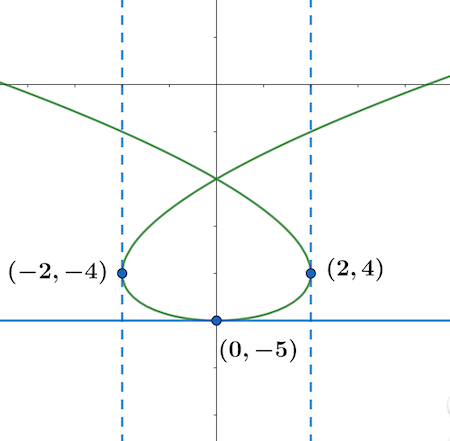# Find the points on the curve where the tangent is horizontal or vertical. If you have a graphing...

## Question:

Find the points on the curve where the tangent is horizontal or vertical. If you have a graphing device, graph the curve to check your work. (Enter your answers as a comma-separated list of ordered pairs.)

{eq}x = t^3 - 3t, y = t^2 - 5 {/eq}

horizontal (x,y) =

vertical tangent (x,y) =

To find the points on the parametric curve {eq}\displaystyle x = t^3-3t, y = t^2-5 {/eq} with horizontal tangent line, we will calculate the slope of the tangent line, as below.

The slope of the tangent line is {eq}\displaystyle \begin{align}\frac{dy}{dx}(t)&=\frac{y'(t)}{x'(t)}=\frac{\dfrac{d}{dt}\left( t^2-5\right)}{\dfrac{d}{dt}\left( t^3-3t\right)}=\frac{2t}{3t^2-3}. \end{align} {/eq}

For the tangent line to be horizontal, we solve the equation given by the slope being zero.

{eq}\displaystyle \begin{align}\frac{dy}{dx}(t)&=0\\ \iff \frac{2t}{3t^2-3}&=0\\ \implies & t=0\\ \iff& \boxed{(x,y) =(0,-5) -\text{ is the point with horizontal tangent line}}. \end{align} {/eq}

For the tangent line to be vertical, we need to have the slope undefined or the denominator to be zero,

{eq}\displaystyle \begin{align}\frac{dy}{dx}(t)&-\text{ undefined}\\ \frac{2t}{3t^2-3}&-\text{ undefined}\\ \implies 3t^2-3&=0\implies t=-1, 1\\ \iff& \boxed{(x,y) =(2,-4) \text{ and } (x,y)=(-2,-4) -\text{ are the points with vertical tangent lines}}. \end{align} {/eq}

The curve is shown below by the solid green line together with the points, obtained above and the corresponding asymptotes.